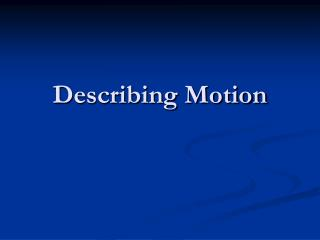Download PresentationDescribing Motion

# Describing Motion - PowerPoint PPT PresentationDownload Presentation## Describing Motion

- - - - - - - - - - - - - - - - - - - - - - - - - - - E N D - - - - - - - - - - - - - - - - - - - - - - - - - - -
##### Presentation Transcript

1. Describing Motion

2. Motion • Sit very still. Do not move. • In what ways are you moving? Changing position?

3. Motion • All motion is relative. • Must be a frame of reference. • MOTION is a change in position of an object with respect to time

4. Motion • How far an object moves is DISTANCE • Meters is the SI unit of length or distance 50 m

5. Motion • DISPLACEMENT is the distance and direction of an objects change in position from the starting point 50 m North

6. Speed • Speed is the distance an object travels per a unit of time. d distance Speed = S = t time

7. Describing Motion • Average speed- total distance traveled divided by total time • It takes me 13 hours to travel 1440 kilometers. My average speed is 110.8 km/hr • Instantaneous speed- speed at a particular point in time, like when you look down at your speedometer. • An object that is speeding up or slowing down, its instantaneous speed is changing

8. Graphing Motion Slope of the line in a distance vs time graph will give you the object’s speed

10. Speed http://www.syossetistops.org/index.php?m=UserPages&e=viewPage&id=1424

11. Velocity • Velocity is the speed and direction of motion of an object in motion. • An up and a down escalator moving at the same speed have different velocities because they are travelling in opposite directions • A car driving in a circle with a constant speed of 100 km/hr has a constantly changing velocity

12. Velocity • These cars are travelling at the same speed. Do they have the same velocity?

13. Velocity • Velocity is the speed and direction of motion of an object in motion. • A change of velocity may be a change of speed. • Or a change of velocity may be constant speed with a change of direction.

14. Practice Problems • A car is moves 20 meters is 4 seconds. What is its speed? • A car is moving 25 m/s for 8 seconds. How far did the car travel?.

15. Practice Problems • A group of bike riders took a 4.0 hour trip. During the first 3.0 hours, they traveled a total of 50. kilometers but during the last hour they traveled only 10. kilometers. What was the group's average speed for the entire trip?

16. Practice Problems • During which interval was the cart at rest?

17. Practice Problems • During which interval was the cart moving in a negative direction?

18. Practice Problems • During which of these two intervals, BC and DE, was the cart traveling at a faster rate?